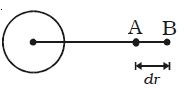Physics

# What is Gravitational Potential?

Gravitational potential at a point is defined as the amount of work done in moving unit mass from the point to infinity against the gravitational field. It is a scalar quantity. Its unit is Nm kg-1

Gravitational potential difference: Gravitational potential difference between two points is defined as the amount of work done in moving unit mass from one point to another point against the gravitational force of attraction. Consider two points A and B separated by a distance dr in the gravitational field.The work done in moving unit mass from A to B is, dv = WA→B

Gravitational potential difference dv = – E dr

Here negative sign indicates that work is done against the gravitational field.